## 对微分代数方程建模

### Robertson 化学反应示例概述

Robertson  创建了一个自催化化学反应方程组来测试和比较刚性方程组的数值求解器。方程组中的化学反应、速率常量 (k) 和反应速率 (V) 如下所示：

`$\begin{array}{ccccc}A& \stackrel{{k}_{1}}{\to }& B& {k}_{1}=0.04& {V}_{1}={k}_{1}\left[A\right]\\ B+B& \stackrel{{k}_{2}}{\to }& C+B& {k}_{2}=3\cdot {10}^{7}& {V}_{2}={k}_{2}\left[B\right]\left[B\right]\\ B+C& \stackrel{{k}_{3}}{\to }& A+C& {k}_{3}=1\cdot {10}^{4}& {V}_{3}={k}_{3}\left[B\right]\left[C\right]\end{array}$`

### 根据 ODE 方程进行 Simulink 建模

• 所有方程均为常微分方程。

• 每个方程都是因变量关于自变量（通常是时间）的导数。

• 方程组中的方程数量等于因变量数量。

`$\begin{array}{l}{A}^{\prime }=-0.04A+1\cdot {10}^{4}BC\\ {B}^{\prime }=0.04A-1\cdot {10}^{4}BC-3\cdot {10}^{7}{B}^{2}\\ {C}^{\prime }=3\cdot {10}^{7}{B}^{2}\end{array}$`

#### 编译模型

1. 将三个 Integrator 模块添加到您的模型中。分别标记输入 `A'``B'``C'` 以及输出 `A``B``C`

2. 添加 Sum、Product 和 Gain 模块，以解算每个微分变量。例如，要对信号 `C'` 建模，

1. 添加一个 Math Function 模块，并将输入信号连接到 `B`。将 Function 参数设置为 “`square`”。

2. 将 Math Function 模块的输出连接到 Gain 模块。将 Gain 参数设置为 `3e7`

3. 继续向您的模型中添加剩余的微分方程项。

3. 通过将 `A` Integrator 模块的 Initial condition 参数设置为 `1`，为 `A` 的初始条件建模。

4. 添加 Out 模块以便将信号 `A``B``C` 保存到 MATLAB 变量 `yout`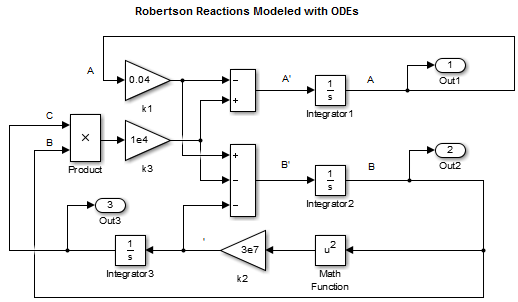#### 对模型进行仿真

1. 在 MATLAB® 脚本中输入以下语句。如果您已构建自己的模型，请将 `ex_hblode` 替换您模型的名称。

```sim('ex_hb1ode') yout(:,2) = 1e4*yout(:,2); figure; semilogx(tout,yout); xlabel('Time'); ylabel('Concentration'); title('Robertson Reactions Modeled with ODEs')```
2. 在 Simulink® Editor 中，在 Modeling 选项卡上，点击 Model Settings

- 在 Solver 窗格中，将 Stop time 设置为 `4e5`，将 Solver 设置 “`ode15s (stiff/NDF)`”。

- 在 Data Import 窗格中，选中 TimeOutput 复选框。

3. 运行脚本。观察是否所有的 `A` 均转换为 `C`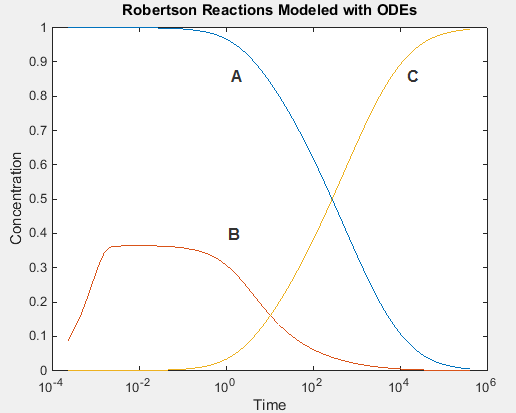### 根据 DAE 方程进行 Simulink 建模

• 它同时包含常微分方程和代数方程。代数方程没有任何导数。

• 只有一些方程是微分方程，它们定义某些因变量的导数。其他因变量使用代数方程定义。

• 方程组中的方程数量等于因变量数量。

`$C=1-A-B$`

`$\begin{array}{l}{A}^{\prime }=-0.04A+1\cdot {10}^{4}BC\\ {B}^{\prime }=0.04A-1\cdot {10}^{4}BC-3\cdot {10}^{7}{B}^{2}\\ C=1-A-B\end{array}$`

#### 编译模型

1. 删除用于计算 `C` 的 Integrator 模块。

2. 添加一个 Sum 模块，并将 List of signs 参数设置为 +– –。

3. 将信号 `A``B` 连接到 Sum 模块的减号输入。

4. `A` 的初始浓度建模，将一个 Constant 模块连接到 Sum 模块的加号输入。将 Constant value 参数设置为 ` 1`

5. 将 Sum 模块的输出连接到分支线，而该分支线连接到 Product 和 Out 模块。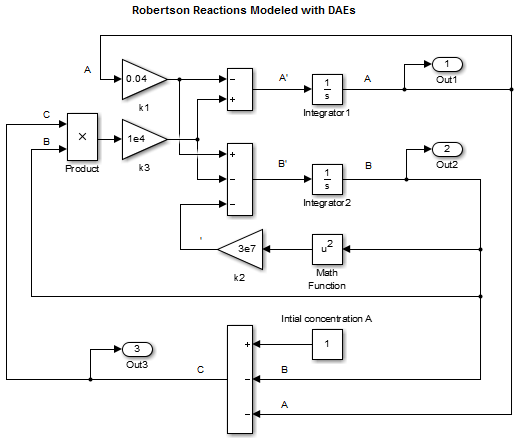#### 对模型进行仿真

1. 在 MATLAB 脚本中输入以下语句。如果您已构建自己的模型，请将 `ex_hbldae` 替换您模型的名称。

```sim('ex_hb1dae') yout(:,2) = 1e4*yout(:,2); figure; semilogx(tout,yout); xlabel('Time'); ylabel('Concentration'); title('Robertson Reactions Modeled with DAEs')```
2. 在 Simulink Editor 中，在 Modeling 选项卡上，点击 Model Settings

- 在 Solver 窗格中，将 Stop time 设置为 `4e5`，将 Solver 设置 “`ode15s (stiff/NDF)`”。

- 在 Data Import 窗格中，选中 TimeOutput 复选框。

3. 运行脚本。使用代数方程时的仿真结果与仅使用微分方程时的模型仿真结果相同。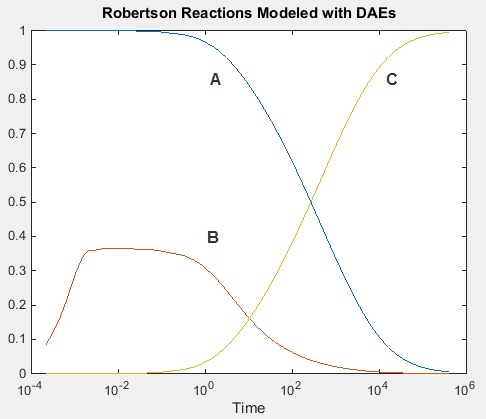### 使用 Algebraic Constraint 模块从 DAE 方程进行 Simulink 建模

`$0=A+B+C-1$`

`$\begin{array}{l}{A}^{\prime }=-0.04A+1\cdot {10}^{4}BC\\ {B}^{\prime }=0.04A-1\cdot {10}^{4}BC-3\cdot {10}^{7}{B}^{2}\\ C=1-A-B\end{array}$`

#### 编译模型

1. 删除用于计算 `C` 的 Integrator 模块。

2. 添加一个 Algebraic Constraint 模块。将 Initial guess 参数设置为 `1e-3`

3. 添加一个 Sum 模块。将 List of signs 参数设置为 –+++。

4. 将信号 `A``B` 连接到 Sum 模块的加号输入。

5. 使用连接到 Sum 模块的减号输入的 Constant 模块对 `A` 的初始浓度建模。将 Constant value 参数设置为 `1`

6. 将 Algebraic Constraint 模块的输出连接到分支线，而该分支线连接到 Product 和 Out 模块输入。

7. 创建一条从 Algebraic Constraint 模块的输出到 Sum 模块的最终加号输入的分支线。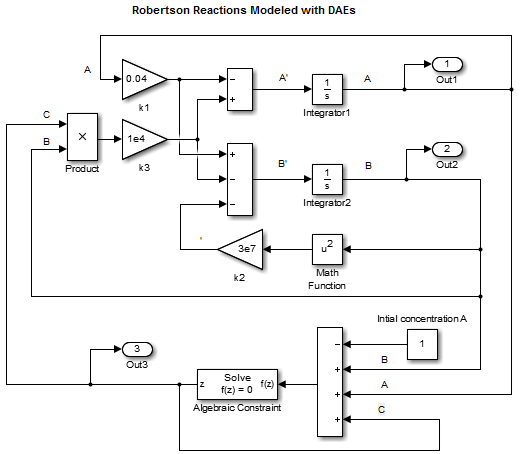#### 对模型进行仿真

1. 在 MATLAB 脚本中输入以下语句。如果您已构建自己的模型，请将 `ex_hbl_acb` 替换您模型的名称。

```sim('ex_hb1dae_acb') yout(:,2) = 1e4*yout(:,2); figure; semilogx(tout,yout); xlabel('Time'); ylabel('Concentration'); title('Robertson Reactions Modeled with DAEs and Algebraic Constraint Block')```
2. 在 Simulink Editor 中，在 Modeling 选项卡上，点击 Model Settings

- 在 Solver 窗格中，将 Stop time 设置为 `4e5`，将 Solver 设置 “`ode15s (stiff/NDF)`”。

- 在 Data Import 窗格中，选中 TimeOutput 复选框。

3. 运行脚本。使用 Algebraic Constraint 模块时的仿真结果与仅使用微分方程时的模型仿真结果相同。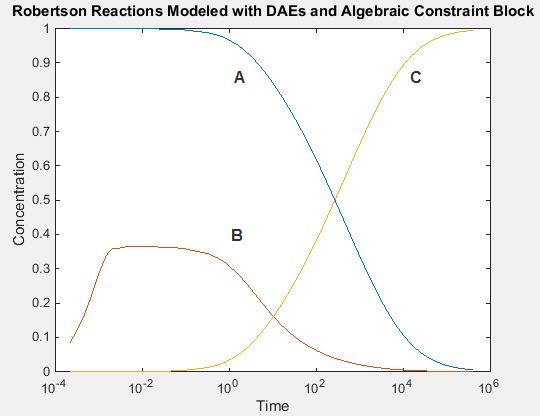Robertson, H. H. “The solution of a set of reaction rate equations.” Numerical Analysis: An Introduction (J. Walsh ed.). London, England:Academic Press, 1966, pp. 178–182.